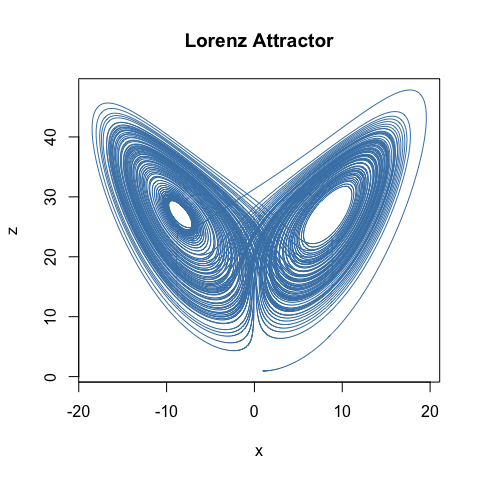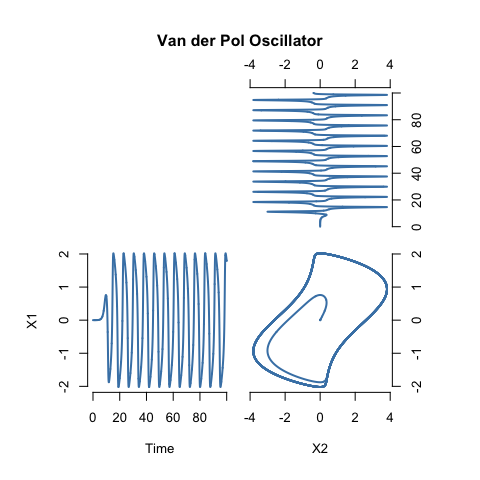# Numerical ODE Solver

odeintr by Timothy H. Keitt is an R package for integrating differential equations with the Boost odeint library. It allows specifying the model in C++ code and compiling it on the fly with Rcpp.

Here we provide an interface to the odeintr package, but

1. Use an intuitive way to specify the ODE model with symengine objects;
2. Use symengine’s code generation functionality to generate the C++ source and compile with odeintr.

However, the interface is not stable and may subject to change in future. This mainly serves an example of how to extend symengine and you are welcome to check out the source code.

library(symengine)
## SymEngine Version: 0.6.0
##  _____           _____         _
## |   __|_ _ _____|   __|___ ___|_|___ ___
## |__   | | |     |   __|   | . | |   | -_|
## |_____|_  |_|_|_|_____|_|_|_  |_|_|_|___|
##       |___|               |___|

## Define ordinary differential equations with symengine

A ordinary differential equation could be constructed with dxdt(x) == rhs where x and rhs will be converted to SymEngine’s ‘Basic’ S4 object. This works by defining a S4 method of == for the return type of dxdt().

For example

x <- Symbol("x")
a <- 3
eq <- dxdt(x) == a/(x + 1)
eq
## Ordinary differential equation:
## d(x)/dt == 3.0/(1.0 + x)

## Define ODE system

ODESystem will take a list of ordinary differential equations, generate C++ source code and compile on the fly with Rcpp. The following is the Lorenz system.

sigma <- 10
rho <- 28
beta <- 8/3
use_vars(x, y, z)
## Initializing 'x', 'y', 'z'
lorenz_sys <- list(
dxdt(x) == sigma * (y - x),
dxdt(y) == (rho - z) * x - y,
dxdt(z) == - beta * z + x * y
)
lorenz_sys <- ODESystem(lorenz_sys, method = "rk5_i")

The method argument is passed to odeintr::compile_sys.

## Get results

A S4 method of predict is defined to run the model with given initial conditions, duration and step_size.

res <- predict(lorenz_sys, init = c(x=1, y=1, z=1),
duration = 100, step_size = 0.001, start = 0)
head(res)
##    Time        x        y         z
## 1 0.000 1.000000 1.000000 1.0000000
## 2 0.001 1.000130 1.025989 0.9983486
## 3 0.002 1.000516 1.051961 0.9967278
## 4 0.003 1.001158 1.077922 0.9951378
## 5 0.004 1.002051 1.103879 0.9935788
## 6 0.005 1.003193 1.129840 0.9920511
plot(res[, c(2, 4)], type = 'l', col = "steelblue", main = "Lorenz Attractor")## Van der Pol Oscillator

Example of Van der Pol oscillator from odeintr package.

use_vars(x, y)
## Initializing 'x', 'y'
vdp_sys <- ODESystem(
dxdt(x) == y,
dxdt(y) == 2 * (1 - x * x) * y - x,
method = "bsd" # Bulirsch-Stoer
)
res <- predict(vdp_sys, init = rep(1e-4, 2), duration = 100, step_size = 0.01)
oldpar <- par(mfrow = c(2, 2), mar = rep(0.5, 4), oma = rep(5, 4), xpd = NA)
make.plot <- function(xy, xlab = NA, ylab = NA)
plot(xy, col = "steelblue", lwd = 2, type = "l",
axes = FALSE, xlab = xlab, ylab = ylab)
plot.new()
make.plot(res[, c(3, 1)]); axis(3); axis(4)
make.plot(res[, c(1, 2)], "Time", "X1"); axis(1); axis(2)
make.plot(res[, c(3, 2)], "X2"); axis(1); axis(4)
title(main = "Van der Pol Oscillator", outer = TRUE)par(oldpar)